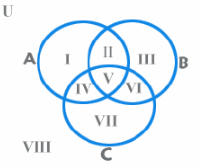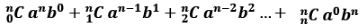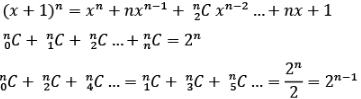Courses

# Important Formulae: Set Theory CAT Notes | EduRev

## Quant : Important Formulae: Set Theory CAT Notes | EduRev

The document Important Formulae: Set Theory CAT Notes | EduRev is a part of the Quant Course Quantitative Aptitude (Quant).
All you need of Quant at this link: Quant

Set EduRev's Tips

The number of elements in a set is called its cardinal number and is written as n(A). A set with cardinal number 0 is called a null set while that with cardinal number ∞ is called an infinite set.
Set A is said to be a subset of Set B if each and every element of Set A is also contained in Set B. Set A is said to be a proper subset of Set B if Set B has at least one element that is not contained in Set A. A set with ‘n’ elements will have 2n subsets (2n – 1 proper subsets)
The Universal set is defined as the set of all possible objects under consideration.

EduRev's Tip: Any set is a subset of itself, but not a proper subset. The empty set, denoted by ∅ , is also a subset of any given set X. The empty set is always a proper subset, except of itself. Every other set is then a subset of the universal set.

Union of two sets is represented as A ∪ B and consists of elements that are present in either Set A or Set B or both. Intersection of two sets is represented as A ∩ B and consists of elements that are present in both Set A and Set B. n(A ∪ B) = n(A) + n(B) — n(A ∩ B)

Venn Diagram: A venn diagram is used to visually represent the relationship between various sets. What do each of the areas in the figure represent?I – only A; II – A and B but not C; III – Only B; IV – A and C but not B; V – A and B and C; VI – B and C but not A; VII – Only C
n(A ∪ B ∪ C) = n(A) + n(B) + n(C) — n(A ∩ B) — n(A ∩ C) -n(B ∩ C) + n(A ∩ B ∩ C)

Binomial Theorem

For some basic values:

• (a + b)° = 1
• (a + b)1 = a + b
• (a + b)2 = a2 + 2ab + b2
• (a + b)3 = a3 + 3a2b + 3ab2 + b3
• (a + b)4 = a+ 4a3b + 6a2b2 + 4ab3 + b4
• (a + b)5 = a5 + 5a4b + 10a3b2 + 10a2b3 + 5ab4 + b5

Theorem
(a + b)n =Some basic properties

EduRev's Tip:

• There is one more term than the power of the exponent, n. That is, there are terms in the expansion of (a + b)n.
• In each term, the sum of the exponents is n, the power to which the binomial is raised.
• The exponents of a start with n, the power of the binomial, and decrease to 0. The last term has no factor of a. The first term has no factor of b, so powers of b start with 0 and increase to n.
• The coefficients start at 1 and increase through certain values about “half”-way and then decrease through these same values back to 1.
• To find the remainder when (x + y)n is divided by x, find the remainder when yn is divided by x.
• (1+x)n ≅ 1 + nx, when x<<1
Offer running on EduRev: Apply code STAYHOME200 to get INR 200 off on our premium plan EduRev Infinity!

## Quantitative Aptitude (Quant)

116 videos|131 docs|131 tests

,

,

,

,

,

,

,

,

,

,

,

,

,

,

,

,

,

,

,

,

,

;